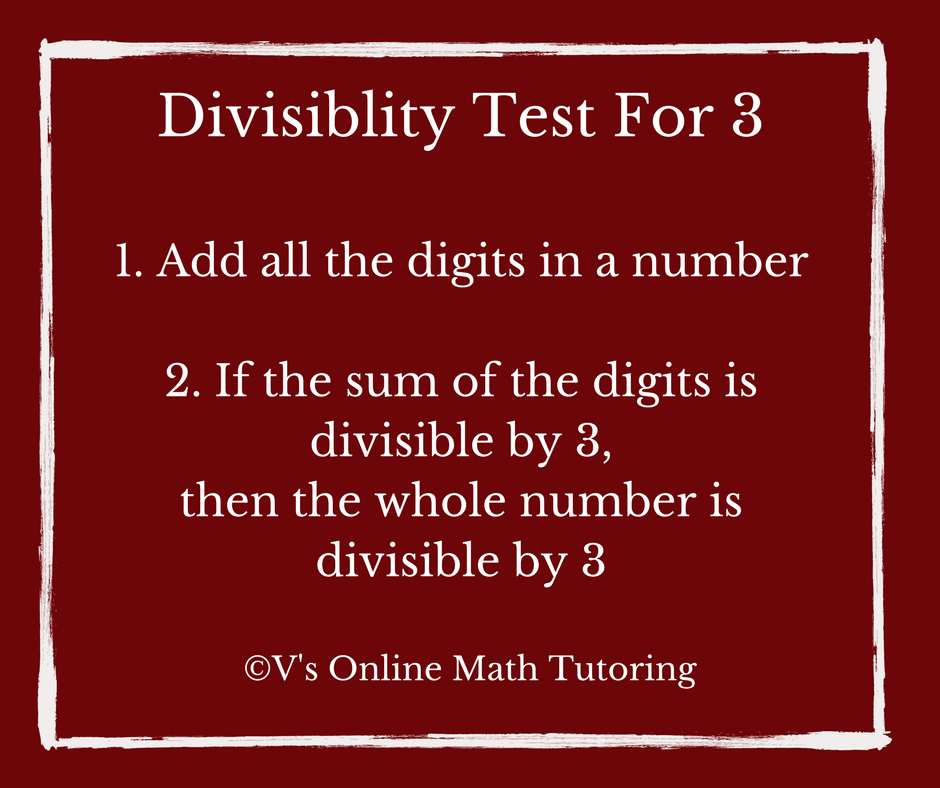top of pageSearch

# Math Competition Problems Problem of the Week - 7

Updated: Jan 22, 2019

The numbers 541A3, 541B3, and 541C3 are all different multiples of 3. The letters A, B, and C represent digits within the numbers. What is the product A × B × C?

Source: MOEMS (Math Olympiad for Elementary and Middle Schools)Math Competition Problems - Problem of the Week 7

## Method 1

To be able to solve this easily, you need to know about divisibility tests. I usually teach my grade 4 or 5 students the divisibility tests once they get reasonably good at problem-solving.

The divisibility test for 3 is as follows:

2. If the sum is divisible by 3, then the whole number is divisible by 3.So take the number given in this problem – 541A3, 541B3, and 541C3.

Add all the digits you have. So, 5+4+1+3 = 13

So after 13 what are the next 3 numbers divisible by 3? 15, 18, 21

So A, B and C can be 15-13=2, 18-13=5, 21-13=8.

Hence A * B * C = 2*5*8 = 80

And the numbers are 54123, 54153 and 54183.## Method 2

For kids who do not know divisibility tests they would solve it by guess and check. This is a fall back method for kids when they have no idea as to how to solve the problem. While this is not the optimal method and takes way too long, this is good when the kid is new to problem-solving.

So here you just plug in various values for A,B and C and sees what works.

For example:

Let A = 1

So we do 54113/3

We get a remainder of (Insert the division picture here)

Then we try A=2

So we do 54123/3

We see that 54123 is divisible by 3. So we take A=2

Then we take B=5 and C=8 and see if that works. After all we can see that even in tens if 30 is divisible by 3, the next ten that is divisible by 3 is 60 and then 90.

Yay! So A=2, B=5 and C=8 works.

Hence A * B * C = 2*5*8 = 80

Note: A, B and C are interchangeable here and you would still end up with the same answer.

Like what you see? Would you like to have the Problem of the Week delivered to your inbox? Click here to subscribe